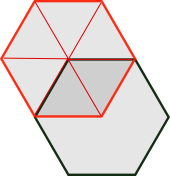#### You may also like### Hallway Borders

What are the possible dimensions of a rectangular hallway if the number of tiles around the perimeter is exactly half the total number of tiles?### Square Pegs

Which is a better fit, a square peg in a round hole or a round peg in a square hole?### Boxed In

A box has faces with areas 3, 12 and 25 square centimetres. What is the volume of the box?

# Overlapping Beer Mats

##### Age 11 to 14 ShortChallenge Level

The top beer mat, outlined in red in the diagram below, can be split into triangles from its centre, as shown below.Because the hexagon is regular, the triangles are all the same, so each one will have area 6 cm$^2$ (since the area of the whole beer mat is 36$=$6$\times$6 cm$^2$).

So, since the overlap consists of 2 of the triangles, its area is 12 cm$^2$.
You can find more short problems, arranged by curriculum topic, in our short problems collection.